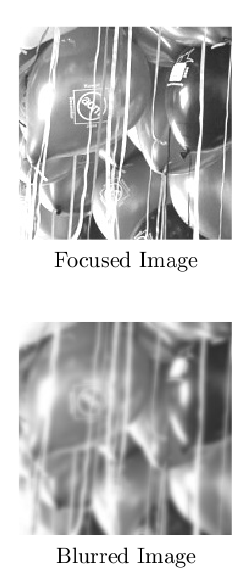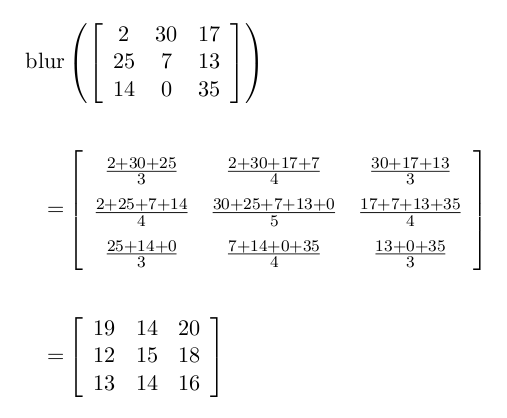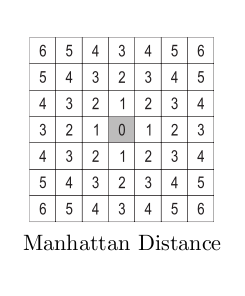## Kind of a blur

View as PDF

Points: 1.31 (partial)
Time limit: 2.17s
Memory limit: 256M
Input: stdin
Output: stdout

Problem source:
ACM ANARC 2009
Problem type
Allowed languages
C, C++, Go, Java, Kotlin, Pascal, PyPy, Python, Rust, ScratchImage blurring occurs when the object being captured is out of the camera's focus. The top two figures on the right are an example of an image and its blurred version. Restoring the original image given only the blurred version is one of the most interesting topics in image processing. This process is called deblurring, which will be your task for this problem.

In this problem, all images are in grey-scale (no colours). Images are represented as a ~2~ dimensional matrix of real numbers, where each cell corresponds to the brightness of the corresponding pixel. Although not mathematically accurate, one way to describe ~a~ blurred image is through averaging all the pixels that are within (less than or equal to) ~a~ certain Manhattan distance† from each pixel (including the pixel itself). Here's an example of how to calculate the blurring of a ~3 \times 3~ image with ~a~ blurring distance of ~1~:Given the blurred version of an image, we are interested in reconstructing the original version assuming that the image was blurred as explained above.

#### Input

Input consists of several test cases. Each case is specified on ~H~ + ~1~ lines.

• The first line specifies three non negative integers specifying the width ~W~, the height ~H~ of the blurred image and the blurring distance ~D~ respectively where ~(1 \leq W~, ~H \leq 10)~ and ~(D \leq min(\frac{W}{2}, \frac{H}{2}))~.
• The remaining ~H~ lines specify the gray-level of each pixel in the blurred image. Each line specifies ~W~ non-negative real numbers given up to the 2nd decimal place. The value of all the given real numbers will be less than ~100~. Zero or more lines (made entirely of white spaces) may appear between cases. The last line of the input file consists of three zeros.

#### Output

For each test case, print a ~W \times H~ matrix of real numbers specifying the deblurred version of the image.

Each element in the matrix should be approximated to ~2~ decimal places. Separate the output of each two consecutive test cases by an empty line. Do not print an empty line after the last test case. It is guaranteed that there is exactly one unique solution for every test case.

#### Sample Input

2 2 1
1 1
1 1
3 3 1
19 14 20
12 15 18
13 14 16
4 4 2
14 15 14 15
14 15 14 15
14 15 14 15
14 15 14 15
0 0 0


#### Sample Output

    1.00    1.00
1.00    1.00

2.00   30.00   17.00
25.00    7.00   13.00
14.00    0.00   35.00

1.00   27.00    2.00   28.00
21.00   12.00   17.00    8.00
21.00   12.00   17.00    8.00
1.00   27.00    2.00   28.00